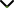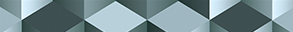# Variable Exponent Function Spaces and their Applications

Publishing date
01 Aug 2021
Status
Closed
26 Mar 2021

Guest Editors

1Hainan Normal University, Haikou, China

2University of M'Sila, M'sila, Algeria

3Northwest Normal University, Lanzhou, China

4Hunan Normal University, Changsha, China

This issue is now closed for submissions.

# Variable Exponent Function Spaces and their Applications

This issue is now closed for submissions.

## DescriptionAs a generalisation of constant Lebesgue spaces, variable exponent Lebegue spaces go back many years, and in subsequent decades variable Lebesgue and Sobolev spaces have been systematically investigated. Since then, many variable exponent real function spaces and complex function spaces have appeared, such as Besov spaces, Trieble-Lizorkin spaces, Hardy spaces, Bessel potential spaces, Herz spaces, Morrey spaces, Herz-Morrey spaces, Bergman spaces, and Fock spaces with variable exponents.

For three decades, variable exponent function spaces have been widely applied in differential equations, image processing, and approximation theory, and many variable exponent real function spaces and complex function spaces have appeared. Thus far, the theory of variable exponent function spaces has seriously depended on the boundedness of the Hardy-Littlewood maximal operator. This restricts its applications in differential equations, approximation, and optimisation.

The aim of this Special Issue is to report developments in theory and applications of variable exponent function spaces, in particular, new variable exponent function spaces, the solvability of differential equations in variable exponent function spaces, the boundedness of operators in variable exponent function spaces, and approximations and optimisations in variable exponent function spaces. We also aim to provide a platform for researchers to promote, share, and discuss various problems in this area. We welcome both original research and review articles.

Potential topics include but are not limited to the following:

• Theory of variable exponent function spaces
• Differential equations in variable exponent function spaces
• Operators on variable exponent function spaces
• Approximations in variable exponent function spaces
• Optimisation in variable exponent function spaces
• Variable exponent function spaces of analytic functionsJournal metrics
See full report
Acceptance rate23%
Submission to final decision72 days
Acceptance to publication20 days
CiteScore2.600
Journal Citation Indicator1.430
Impact Factor1.9Author guidelinesEditorial boardDatabases and indexing

Article of the Year Award: Impactful research contributions of 2022, as selected by our Chief Editors. Discover the winning articles.